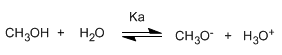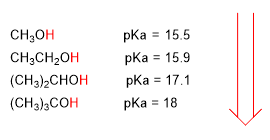Alcohols are amphoteric (amphiprotic) species, they can act as acids or bases. In aqueous solution an equilibrium is established between alcohol, water and their conjugate bases.Writing the equilibrium constant (Ka)The small value of the constant tells us that the equilibrium is fully shifted to the left. The changed logarithm of the equilibrium constant gives us the pKa of methanol, a parameter that indicates the degree of acidity of an organic compound.

pKa = - log ka = 15.5

An increase in pKa implies a decrease in acidity. Thus, methanol with a pka of 15.5 is slightly more acidic than ethanol with a pka of 15.9.

The pKa of alcohols is influenced by some factors such as the size of the carbon chain and the electronegative groups.

As the carbon chain length increases, the alcohol becomes less acidic.lower acidity

Electronegative groups (halogens) increase the acidity of alcohols (lower the pKa)higher acidity